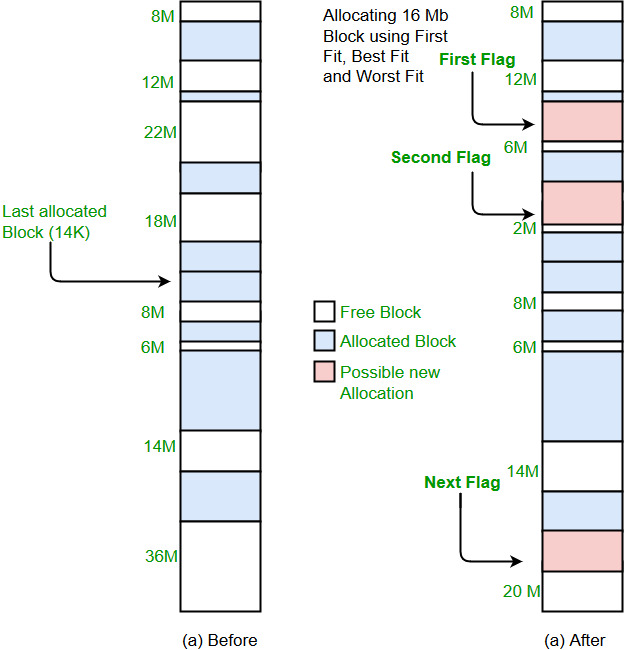Related Articles

# Program for Worst Fit algorithm in Memory Management

• Difficulty Level : Easy
• Last Updated : 28 Jun, 2021

Prerequisite : Partition allocation methods
Worst Fit allocates a process to the partition which is largest sufficient among the freely available partitions available in the main memory. If a large process comes at a later stage, then memory will not have space to accommodate it.

Example:

```Input : blockSize[]   = {100, 500, 200, 300, 600};
processSize[] = {212, 417, 112, 426};
Output:
Process No.    Process Size    Block no.
1        212        5
2        417        2
3        112        5
4        426        Not Allocated``````Implementation:
1- Input memory blocks and processes with sizes.
2- Initialize all memory blocks as free.
3- Start by picking each process and find the
maximum block size that can be assigned to
current process i.e., find max(bockSize,
blockSize,.....blockSize[n]) >
processSize[current], if found then assign
it to the current process.
5- If not then leave that process and keep checking
the further processes.```

Below is implementation of above steps.

## C++

 `// C++ implementation of worst - Fit algorithm``#include``using` `namespace` `std;` `// Function to allocate memory to blocks as per worst fit``// algorithm``void` `worstFit(``int` `blockSize[], ``int` `m, ``int` `processSize[],``                                                 ``int` `n)``{``    ``// Stores block id of the block allocated to a``    ``// process``    ``int` `allocation[n];` `    ``// Initially no block is assigned to any process``    ``memset``(allocation, -1, ``sizeof``(allocation));` `    ``// pick each process and find suitable blocks``    ``// according to its size ad assign to it``    ``for` `(``int` `i=0; i= processSize[i])``            ``{``                ``if` `(wstIdx == -1)``                    ``wstIdx = j;``                ``else` `if` `(blockSize[wstIdx] < blockSize[j])``                    ``wstIdx = j;``            ``}``        ``}` `        ``// If we could find a block for current process``        ``if` `(wstIdx != -1)``        ``{``            ``// allocate block j to p[i] process``            ``allocation[i] = wstIdx;` `            ``// Reduce available memory in this block.``            ``blockSize[wstIdx] -= processSize[i];``        ``}``    ``}` `    ``cout << ``"\nProcess No.\tProcess Size\tBlock no.\n"``;``    ``for` `(``int` `i = 0; i < n; i++)``    ``{``        ``cout << ``"   "` `<< i+1 << ``"\t\t"` `<< processSize[i] << ``"\t\t"``;``        ``if` `(allocation[i] != -1)``            ``cout << allocation[i] + 1;``        ``else``            ``cout << ``"Not Allocated"``;``        ``cout << endl;``    ``}``}` `// Driver code``int` `main()``{``    ``int` `blockSize[] = {100, 500, 200, 300, 600};``    ``int` `processSize[] = {212, 417, 112, 426};``    ``int` `m = ``sizeof``(blockSize)/``sizeof``(blockSize);``    ``int` `n = ``sizeof``(processSize)/``sizeof``(processSize);` `    ``worstFit(blockSize, m, processSize, n);` `    ``return` `0 ;``}`

## Java

 `// Java implementation of worst - Fit algorithm` `public` `class` `GFG``{``    ``// Method to allocate memory to blocks as per worst fit``    ``// algorithm``    ``static` `void` `worstFit(``int` `blockSize[], ``int` `m, ``int` `processSize[],``                                                     ``int` `n)``    ``{``        ``// Stores block id of the block allocated to a``        ``// process``        ``int` `allocation[] = ``new` `int``[n];``     ` `        ``// Initially no block is assigned to any process``        ``for` `(``int` `i = ``0``; i < allocation.length; i++)``            ``allocation[i] = -``1``;``     ` `        ``// pick each process and find suitable blocks``        ``// according to its size ad assign to it``        ``for` `(``int` `i=``0``; i= processSize[i])``                ``{``                    ``if` `(wstIdx == -``1``)``                        ``wstIdx = j;``                    ``else` `if` `(blockSize[wstIdx] < blockSize[j])``                        ``wstIdx = j;``                ``}``            ``}``     ` `            ``// If we could find a block for current process``            ``if` `(wstIdx != -``1``)``            ``{``                ``// allocate block j to p[i] process``                ``allocation[i] = wstIdx;``     ` `                ``// Reduce available memory in this block.``                ``blockSize[wstIdx] -= processSize[i];``            ``}``        ``}``     ` `        ``System.out.println(``"\nProcess No.\tProcess Size\tBlock no."``);``        ``for` `(``int` `i = ``0``; i < n; i++)``        ``{``            ``System.out.print(``"   "` `+ (i+``1``) + ``"\t\t"` `+ processSize[i] + ``"\t\t"``);``            ``if` `(allocation[i] != -``1``)``                ``System.out.print(allocation[i] + ``1``);``            ``else``                ``System.out.print(``"Not Allocated"``);``            ``System.out.println();``        ``}``    ``}``    ` `    ``// Driver Method``    ``public` `static` `void` `main(String[] args)``    ``{``         ``int` `blockSize[] = {``100``, ``500``, ``200``, ``300``, ``600``};``         ``int` `processSize[] = {``212``, ``417``, ``112``, ``426``};``         ``int` `m = blockSize.length;``         ``int` `n = processSize.length;``         ` `         ``worstFit(blockSize, m, processSize, n);``    ``}``}`

## Python3

 `# Python3 implementation of worst - Fit algorithm` `# Function to allocate memory to blocks as``# per worst fit algorithm``def` `worstFit(blockSize, m, processSize, n):``    ` `    ``# Stores block id of the block``    ``# allocated to a process``    ` `    ``# Initially no block is assigned``    ``# to any process``    ``allocation ``=` `[``-``1``] ``*` `n``    ` `    ``# pick each process and find suitable blocks``    ``# according to its size ad assign to it``    ``for` `i ``in` `range``(n):``        ` `        ``# Find the best fit block for``        ``# current process``        ``wstIdx ``=` `-``1``        ``for` `j ``in` `range``(m):``            ``if` `blockSize[j] >``=` `processSize[i]:``                ``if` `wstIdx ``=``=` `-``1``:``                    ``wstIdx ``=` `j``                ``elif` `blockSize[wstIdx] < blockSize[j]:``                    ``wstIdx ``=` `j` `        ``# If we could find a block for``        ``# current process``        ``if` `wstIdx !``=` `-``1``:``            ` `            ``# allocate block j to p[i] process``            ``allocation[i] ``=` `wstIdx` `            ``# Reduce available memory in this block.``            ``blockSize[wstIdx] ``-``=` `processSize[i]` `    ``print``(``"Process No. Process Size Block no."``)``    ``for` `i ``in` `range``(n):``        ``print``(i ``+` `1``, ``"         "``,``              ``processSize[i], end ``=` `"     "``)``        ``if` `allocation[i] !``=` `-``1``:``            ``print``(allocation[i] ``+` `1``)``        ``else``:``            ``print``(``"Not Allocated"``)` `# Driver code``if` `__name__ ``=``=` `'__main__'``:``    ``blockSize ``=` `[``100``, ``500``, ``200``, ``300``, ``600``]``    ``processSize ``=` `[``212``, ``417``, ``112``, ``426``]``    ``m ``=` `len``(blockSize)``    ``n ``=` `len``(processSize)` `    ``worstFit(blockSize, m, processSize, n)` `# This code is contributed by PranchalK`

## C#

 `// C# implementation of worst - Fit algorithm``using` `System;` `class` `GFG``{``    ``// Method to allocate memory to blocks ``    ``// as per worst fit algorithm``    ``static` `void` `worstFit(``int` `[]blockSize, ``int` `m,``                        ``int` `[]processSize, ``int` `n)``    ``{``        ``// Stores block id of the block allocated to a``        ``// process``        ``int` `[]allocation = ``new` `int``[n];``    ` `        ``// Initially no block is assigned to any process``        ``for` `(``int` `i = 0; i < allocation.Length; i++)``            ``allocation[i] = -1;``    ` `        ``// pick each process and find suitable blocks``        ``// according to its size ad assign to it``        ``for` `(``int` `i = 0; i < n; i++)``        ``{``            ``// Find the best fit block for current process``            ``int` `wstIdx = -1;``            ``for` `(``int` `j = 0; j < m; j++)``            ``{``                ``if` `(blockSize[j] >= processSize[i])``                ``{``                    ``if` `(wstIdx == -1)``                        ``wstIdx = j;``                    ``else` `if` `(blockSize[wstIdx] < blockSize[j])``                        ``wstIdx = j;``                ``}``            ``}``    ` `            ``// If we could find a block for current process``            ``if` `(wstIdx != -1)``            ``{``                ``// allocate block j to p[i] process``                ``allocation[i] = wstIdx;``    ` `                ``// Reduce available memory in this block.``                ``blockSize[wstIdx] -= processSize[i];``            ``}``        ``}``    ` `        ``Console.WriteLine(``"\nProcess No.\tProcess Size\tBlock no."``);``        ``for` `(``int` `i = 0; i < n; i++)``        ``{``            ``Console.Write(``" "` `+ (i+1) + ``"\t\t\t"` `+ processSize[i] + ``"\t\t\t"``);``            ``if` `(allocation[i] != -1)``                ``Console.Write(allocation[i] + 1);``            ``else``                ``Console.Write(``"Not Allocated"``);``            ``Console.WriteLine();``        ``}``    ``}``    ` `    ``// Driver code``    ``public` `static` `void` `Main(String[] args)``    ``{``        ``int` `[]blockSize = {100, 500, 200, 300, 600};``        ``int` `[]processSize = {212, 417, 112, 426};``        ``int` `m = blockSize.Length;``        ``int` `n = processSize.Length;``        ` `        ``worstFit(blockSize, m, processSize, n);``    ``}``}` `// This code has been contributed by 29AjayKumar`

## Javascript

 ``

Output:

```Process No.    Process Size    Block no.
1        212        5
2        417        2
3        112        5
4        426        Not Allocated```

This article is contributed by Sahil Chhabra (akku). If you like GeeksforGeeks and would like to contribute, you can also write an article using write.geeksforgeeks.org or mail your article to review-team@geeksforgeeks.org. See your article appearing on the GeeksforGeeks main page and help other Geeks.